# Inductor Mathematics

For March 2016, our friends at Keysight Technologies gave us a video on how to model spiral inductors.  Thanks, Keysight!

Below is an index of our mathematical discussion of inductors:

Inductive reactance

Inductance of a transmission line (separate page)

Solenoid inductance

Spiral inductor (wire)

Spiral inductors on a substrate (New for March 2016!)

Toroid inductance

Wirebond inductance (now on a separate page)

Airbridge inductance (separate page)

Via hole inductance (separate page)

Wirebond inductance (separate page)

DC and RF resistance of inductors

Resonances

### Inductive reactance

Use our reactance calculator if you are interested in this topic!

The well-known equation for inductive reactance is shown below. Note that inductive reactance is positive, the opposite polarity of capacitive reactance. On the Smith Chart, this means that series inductance tends to move a reflection coefficient in a clockwise direction.

###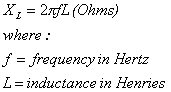A more useful form of the inductive reactance equation is given below, where frequency is in GHz and inductance is in nanohenries. Luckily all of those decimal places just cancel each other out!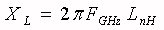### Solenoid inductance

A solenoid is a cylindrical shape that is wound with wire to create inductance. It can have a single layer of windings, or multilayer, and it can use an air core or a core with high magnetic permeability for increased inductance. The most useful (read that "highest Q") solenoids for microwave applications are miniature, single-layer air-core inductors. The graphic below was contributed by Sebastiaan. Many thanks!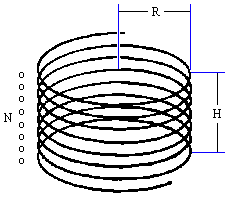The classic formula for single-layer inductance (air core) is called Wheeler's formula, which dates back to the radio days of the 1920s: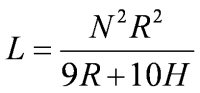where:

L = inductance in micro-Henries (not nano-Henries!)
N= number of turns of wire
R= radius of coil in inches
H= height of coil in inches

Here it is in terms of D, the diameter of the coil: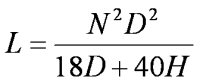(This formula was corrected April 9, 2006 thanks to KB!)

Wheeler's formula does not take into account wire diameter, and spacing between the turns. In the Wheeler formula, the turns are touching each other, but some insulation is assumed to prevent shorting out. In practice, some spacing between turns is necessary to reduce the inter-turn capacitance and increase the operating frequency. Let's face it, Wheeler was not interested in the accuracy of nano-Henry coils for microwave hardware.

A supposedly more-accurate method of calculating inductance of single-layer air-core inductors for microwave components can be found on Microwave Components Incorporated web site: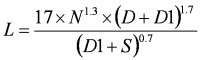where:

L = inductance in nano-Henries
N = number of turns of wire
D = inside diameter of the coil (inches)
D1 = bare wire diameter (inches)
S = space between turns (inches)

Using the MCI formula, applied to 47 gauge wire (1.2 mil bare wire diameter), and 0.5 mil spacing between turns, wrapping the turns around a 20 mil pin vice, you can make the following air-coil values:

1 turn= 2 nH
2 turns= 5 nH
3 turns = 8 nH
4 turns = 12 nH
5 turns= 16 nH
6 turns = 20 nH
7 turns = 25 nH
8 turns= 30 nH
9 turns = 35 nH
10 turns = 40 nH

### Spiral inductor (wire)

This formula and graphic was also contributed by Sebastian (units are also micro-Henries): We have to admit, we haven't personally tested some formulas on this page for accuracy against measured data. Also, note that any inductor model that doesn't consider parasitic capacitance and resistance will have limited accuracy at microwave frequencies.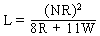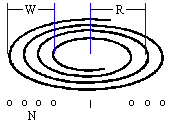### Spiral inductors on a substrate

Spiral inductors are often used in MIC, MMIC and RFIC design, particularly below 18 GHz. Inductors can be rectangular or round, so long as you know how to model them. The spiral inductor lumped element model can have many capacitor and resistor elements to account for all the parasitics that make it less and less ideal as you move up in frequency.  Modeling an inductor requires good de-embedded data on one or more inductor values, which result in a scalable model that allows you to predict the characteristics of arbitrary inductor values required by your design.

Want a little more hands-on description of how to model spiral inductors?  Check out this video on EXACTLY THAT. Franz Sischka of SisConsult takes you through a complete lumped element model of a spiral inductor, including skin effects, substrate eddy currents, and coupling to metal 1 shielding. Keysight's Integrated Circuit Characterization and Analysis Program (IC-CAP) is used to fit two example measurements. The elements are manually tuned followed by optimization. Methods of model verification are provided and the example files can be downloaded.

### Toroid inductance

A toroid is similar to a solenoid, but is donut shaped. More to come!

### DC and RF resistance of inductors

Computing the DC resistance of a spiral inductor is simple, and is often overlooked by designers until they build an amplifier circuit and the part doesn't bias up correctly on the first iteration. You need to know the sheet resistance of your metalization, in ohms per square, and compute the number of squares in the inductor. The number of squares is the total length (if you unwound it) divided by the width, and can easily run into the hundreds for a large inductor.

Computing the RF resistance, you may have to consider the skin depth effect.

The model shown below is the classic model for spiral inductors. Computing the elements is not as straightforward as you might hope.

### Inductor resonances

More to come!

Author : Unknown Editor